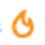$Q1$ Find the largest y such that

$\frac { 1 }{ 1+{ x }^{ 2 } } \ge \frac { y-x }{ y+x } \quad for\quad all\quad x>0$

$Q2$ Find the minimum and maximum values of

$\frac { x+1 }{ xy+x+1 } +\frac { y+1 }{ yz+y+1 } +\frac { z+1 }{ zx+z+1 }$Note by Satyajit Ghosh
5 years, 8 months ago

This discussion board is a place to discuss our Daily Challenges and the math and science related to those challenges. Explanations are more than just a solution — they should explain the steps and thinking strategies that you used to obtain the solution. Comments should further the discussion of math and science.

When posting on Brilliant:

• Use the emojis to react to an explanation, whether you're congratulating a job well done , or just really confused .
• Ask specific questions about the challenge or the steps in somebody's explanation. Well-posed questions can add a lot to the discussion, but posting "I don't understand!" doesn't help anyone.
• Try to contribute something new to the discussion, whether it is an extension, generalization or other idea related to the challenge.

MarkdownAppears as
*italics* or _italics_ italics
**bold** or __bold__ bold
- bulleted- list
• bulleted
• list
1. numbered2. list
1. numbered
2. list
Note: you must add a full line of space before and after lists for them to show up correctly
paragraph 1paragraph 2

paragraph 1

paragraph 2

[example link](https://brilliant.org)example link
> This is a quote
This is a quote
    # I indented these lines
# 4 spaces, and now they show
# up as a code block.

print "hello world"
# I indented these lines
# 4 spaces, and now they show
# up as a code block.

print "hello world"
MathAppears as
Remember to wrap math in $$ ... $$ or $ ... $ to ensure proper formatting.
2 \times 3 $2 \times 3$
2^{34} $2^{34}$
a_{i-1} $a_{i-1}$
\frac{2}{3} $\frac{2}{3}$
\sqrt{2} $\sqrt{2}$
\sum_{i=1}^3 $\sum_{i=1}^3$
\sin \theta $\sin \theta$
\boxed{123} $\boxed{123}$

Sort by:

- 5 years, 8 months ago

@Satyajit Ghosh Thank you for mentioning me!

- 5 years, 8 months ago

Applying componendo and dividendo we get $\frac{2+x^{2}}{-x^{2}}\geq\frac{2y}{-2x}$

$\frac{2}{x}+x \geq y$

Applying $A.M-G.M$

$\frac{2}{x}+x\geq 2\sqrt{2}$

Therefore $y=2\sqrt{2}$.

- 5 years, 8 months ago

Thanks. How could I forget to tag you! Btw can you even have a look at Olympiad corner#1 q1

- 5 years, 8 months ago

- 5 years, 8 months ago

He is asking for question 1 solution. 2nd was easy.

- 5 years, 8 months ago

- 5 years, 8 months ago

Lol both questions are Q1?

- 5 years, 8 months ago

I've fixed it.

- 5 years, 8 months ago

Do u think the 2nd question is correct? Check the expression again from your book.

- 5 years, 8 months ago

Sorry the denominator of 1st term had xy+y+1 but now I have updated it it to the correct question

- 5 years, 8 months ago

If you have got the answer, please give a hint at least

- 5 years, 8 months ago

1. Cross multiplying we get $\dfrac{y+x}{y-x} \ge 1+x^{2}$ or $\dfrac{2}{y-x} \ge x$ or $x^{2}-yx+2 \le 0$.
This is a quadratic equation in $x$ and since it is $\le 0$, the discriminant i.e. $y^{2}-8$ must also be $\le 0$ or $y \le \sqrt {8}$.

Therefore, the maximum value of $y$ is $\sqrt {8}$

- 5 years, 8 months ago

Thanks! Can you tell the answer for q2. Do check out my Olympiad corner#1 which has q1 unanswered.

- 5 years, 8 months ago